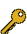Last Update: 2023-04-01 07:05:47

## 6-f. \$B>pJs=hM}5;=Q(B

\$B:G=*99?7F|;~!'(B2021-08-21 18:44:01\$B\$3\$NJ,N`\$G\$h\$/;H\$o\$l(B
\$B\$F\$\$\$k%-!<%o!<%I(B
\$B%-!<%o!<%I(B\$B
Design Space1\$B7o(BOptimization1\$B7o(BContinuous Manufacturing1\$B7o(BStatistical modeling1\$B7o(BModeling1\$B7o(BAutomatic physical model building1\$B7o(BQuality by Design1\$B7o(BProcess systems engineering1\$B7o(B\$B\$BHV9f(B\$B9V1iBjL\!?H/I=\$B%-!<%o!<%I(B\$BH/I=7A<0(B
586\$B?t<072\$+\$iJ*M}%b%G%k\$N8uJd\$r<+F0\$G=PNO\$9\$k%"%k%4%j%:%`\$N3+H/(B
(\$B5~Bg1!>pJs(B) \$B!{(B(\$B3X(B)\$B2CF#(B \$B>MB@(B\$B!&(B (\$B@5(B)\$B2CG<(B \$B3X(B
Automatic physical model building
Modeling
Process systems engineering
O
638\$BB?9)Dx@8;:%7%9%F%`\$K\$*\$1\$kJbN1\$jM=B,\$N\$?\$a\$N=EMW9)DxA*Br(B
(\$B5~Bg1!>pJs(B) \$BCv;t(B \$BH;8c(B\$B!&(B \$B!{(B(\$B@5(B)\$B2CG<(B \$B3X(B
Statistical modeling
Optimization
O
640\$B0eLtIJO"B3@8;:\$rBP>]\$H\$7\$?%G%6%\$%s%9%Z!<%9\$N@_7W(B
(\$B5~Bg1!>pJs(B) \$BB\$B!&(B \$B!{(B(\$B@5(B)\$B2CG<(B \$B3X(B
Quality by Design
Continuous Manufacturing
Design Space
O

\$B9V1i?=\$79~\$_0lMw(B(\$B%7%s%]%8%&%`HV9f!&9V1iJ,N`HV9fJL(B)

\$B

This page was generated byeasp 2.46; update.pl 2.37 (C)1999-2015 kawase